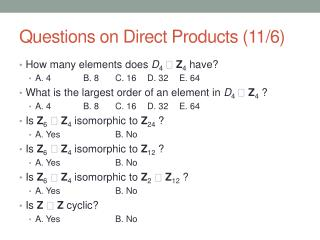DownloadDownload PresentationQuestions on Direct Products (11/6)

# Questions on Direct Products (11/6)

Télécharger la présentation## Questions on Direct Products (11/6)

- - - - - - - - - - - - - - - - - - - - - - - - - - - E N D - - - - - - - - - - - - - - - - - - - - - - - - - - -
##### Presentation Transcript

1. Questions on Direct Products (11/6) • How many elements does D4  Z4 have? • A. 4 B. 8 C. 16 D. 32 E. 64 • What is the largest order of an element in D4  Z4? • A. 4 B. 8 C. 16 D. 32 E. 64 • Is Z6 Z4isomorphic to Z24 ? • A. Yes B. No • Is Z6 Z4 isomorphic to Z12 ? • A. Yes B. No • Is Z6  Z4 isomorphic to Z2 Z12 ? • A. Yes B. No • Is Z  Z cyclic? • A. Yes B. No

2. More Questions • What is the least number of factors possible to express a group which is isomorphic to Z4 Z3  Z7 ? • A. 1 B. 2 C. 3 (Write down the group.) • What is the least number of factors possible to express a group which is isomorphic to Z2 Z2  Z3  Z7 ? • A. 1 B. 2 C. 3 D. 4 (Write down the group.) • What is the least number of factors possible to express a group which is isomorphic to Z2 Z8  Z3  Z9  Z5  Z5? • A. 1 B. 2 C. 3 D. 4 E. 6 (Write down “the” group.) • What is the least number of factors possible to express a group which is isomorphic to Z24 Z26? • A. 1 B. 2 (Write down “the” group.)

3. Questions on U-groups • U(19) is isomorphic to: • A. Z19 B. Z18 C. Z2 Z9 • U(16) is isomorphic to: • A. Z16 B. Z8 C. Z2 Z4 D. Z2 Z2 Z2 • U(20) is isomorphic to: • A. Z20 B. Z8 C. Z2 Z4 D. Z2 Z2 Z2 • U(26) is cyclic. • A. True B. False • U(143) is cyclic. • A. True B. False

4. A Fundamental Theorem! • The Fundamental Theorem of Finite Abelian Groups.Every finite abelian group G is isomorphic to an external direct product of cyclic groups. In particular, G can be written in a unique way (up to arrangement of the factors) as a product of cyclic groups of prime power order. • Note the strong similarity to the Fundamental Theorem of Arithmetic! In fact, if G is itself cyclic, it really is the same. • Proof is given (at some length!) in Chapter 11. We omit it. • Corollary. The converse of Lagrange’s Theorem holds for all abelian groups (since it holds for all cyclic groups). That is, if G is abelian of order n and if k divides n, then G has at least one subgroup of order k.

5. Two Common Forms & Examples • Example. Write Z60 in “prime power form”. Note that this is exactly like the Fundamental Theorem of Arithmetic applied to 60. • Example. Write Z2 Z30 in prime power form. • These two groups were given to us in the other very useful form, called “least factors with divisibility.” This form is nice because it’s simplest and because you can read off directly the largest order of the elements. This form is also unique (even in the arrangement) for a given group. • Example. G, in prime-power form, is isomorphic to Z4 Z3  Z11. Write it in least factors w/ div form. • Example. Same for Z2 Z8  Z3  Z9  Z5  Z5.

6. Assignment for Friday • Be sure the reading and the exercises assigned last time in Chapter 8 are done and add Exercises 52, 53, 70, 71, 72, 73. • Write down, in both prime power form and least factors with divisibility form, all abelian groups of order: • a. 105 b. 140 c. 360 (there are 6 such groups!)